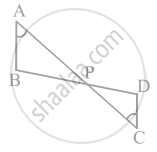# In figure, if ∠A = ∠C, AB = 6 cm, BP = 15 cm, AP = 12 cm and CP = 4 cm, then find the lengths of PD and CD. - Mathematics

Sum

In figure, if ∠A = ∠C, AB = 6 cm, BP = 15 cm, AP = 12 cm and CP = 4 cm, then find the lengths of PD and CD.#### Solution

Given

∠A = ∠C,

AB = 6 cm, BP = 15 cm,

AP = 12 cm

CP = 4 cm

From ∆APB and ∆CPD,

∠A = ∠C

∠APB = ∠CPD  .....[Vertically opposite angles]

∴ By AAA similarity criteria,

ΔAPD ∼ ΔCPD

Using basic proportionality theorem,

⇒ (AP)/(CP) = (PB)/(PD) = (AB)/(CD)  ......[By basic proportionality theorem]

⇒ 12/4 = 15/(PD) = 6/(CD)

Considering (AP)/(CP) = (PB)/(PD), we get,

12/4 = 15/(PD)

PD = (15 xx 4)/12 = 60/12 = 5 cm

Considering, (AP)/(CP) = (AB)/(CD)

⇒ CD = ((6 xx 4))/12 = 2 cm

Therefore,

Length of PD = 5 cm

Length of CD = 2 cm

Concept: Basic Proportionality Theorem (Thales Theorem)
Is there an error in this question or solution?

#### APPEARS IN

NCERT Mathematics Exemplar Class 10
Chapter 6 Triangles
Exercise 6.4 | Q 1 | Page 73

Share